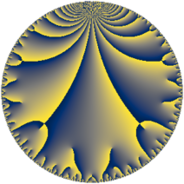Properties

 Label 42.9.hLevel $42$ Weight $9$ Character orbit 42.h Rep. character $\chi_{42}(11,\cdot)$ Character field $\Q(\zeta_{6})$ Dimension $44$ Newform subspaces $1$ Sturm bound $72$ Trace bound $0$

Related objects

Defining parameters

 Level: $$N$$ $$=$$ $$42 = 2 \cdot 3 \cdot 7$$ Weight: $$k$$ $$=$$ $$9$$ Character orbit: $$[\chi]$$ $$=$$ 42.h (of order $$6$$ and degree $$2$$) Character conductor: $$\operatorname{cond}(\chi)$$ $$=$$ $$21$$ Character field: $$\Q(\zeta_{6})$$ Newform subspaces: $$1$$ Sturm bound: $$72$$ Trace bound: $$0$$

Dimensions

The following table gives the dimensions of various subspaces of $$M_{9}(42, [\chi])$$.

Total New Old
Modular forms 136 44 92
Cusp forms 120 44 76
Eisenstein series 16 0 16

Trace form

 $$44 q + 2816 q^{4} + 512 q^{6} + 7350 q^{7} + 11164 q^{9} + O(q^{10})$$ $$44 q + 2816 q^{4} + 512 q^{6} + 7350 q^{7} + 11164 q^{9} - 5888 q^{10} - 89164 q^{13} + 145084 q^{15} - 360448 q^{16} + 16896 q^{18} - 326582 q^{19} + 18394 q^{21} - 40960 q^{22} + 32768 q^{24} + 1923042 q^{25} + 1026900 q^{27} + 520704 q^{28} - 531968 q^{30} + 2693270 q^{31} - 4167178 q^{33} + 1826816 q^{34} + 2857984 q^{36} - 2947454 q^{37} + 2333410 q^{39} + 753664 q^{40} - 5799168 q^{42} - 14383244 q^{43} - 11444734 q^{45} - 10628608 q^{46} + 13896206 q^{49} + 17787386 q^{51} - 5706496 q^{52} + 1193984 q^{54} - 11908144 q^{55} + 89549280 q^{57} + 7485184 q^{58} + 9285376 q^{60} + 72136904 q^{61} - 88725144 q^{63} - 92274688 q^{64} - 31015936 q^{66} - 51651510 q^{67} - 22794652 q^{69} + 118232576 q^{70} - 2162688 q^{72} + 134471754 q^{73} - 160265882 q^{75} - 83604992 q^{76} + 69060608 q^{78} - 31207594 q^{79} + 126111196 q^{81} + 66594816 q^{82} - 56941568 q^{84} - 280800088 q^{85} - 70024916 q^{87} - 2621440 q^{88} + 141565952 q^{90} + 130228838 q^{91} - 82315016 q^{93} + 108564480 q^{94} - 4194304 q^{96} + 424892352 q^{97} + 562622116 q^{99} + O(q^{100})$$

Decomposition of $$S_{9}^{\mathrm{new}}(42, [\chi])$$ into newform subspaces

Label Dim. $$A$$ Field CM Traces $q$-expansion
$a_{2}$ $a_{3}$ $a_{5}$ $a_{7}$
42.9.h.a $44$ $17.110$ None $$0$$ $$0$$ $$0$$ $$7350$$

Decomposition of $$S_{9}^{\mathrm{old}}(42, [\chi])$$ into lower level spaces

$$S_{9}^{\mathrm{old}}(42, [\chi]) \cong$$ $$S_{9}^{\mathrm{new}}(21, [\chi])$$$$^{\oplus 2}$$